# Hard superball model

(diff) ← Older revision | Latest revision (diff) | Newer revision → (diff)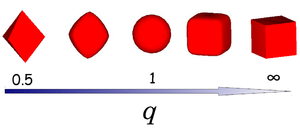The shape of superballs interpolates between octahedra (q = 0.5) and cubes (q = ∞) via spheres (q = 1).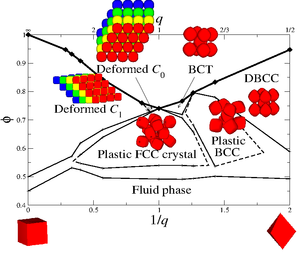Phase diagram for hard superballs in the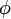$\phi$ (packing fraction) versus 1/q (bottom axis) and q (top axis) representation where q is the deformation parameter .

The hard superball model is defined by the inequality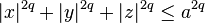$|x|^{2q} + |y|^{2q} +|z|^{2q} \le a^{2q}$

where x, y and z are scaled Cartesian coordinates with q the deformation parameter and radius a. The shape of the superball interpolates smoothly between two Platonic solids, namely the octahedron (q = 0.5) and the cube (q = ∞) via the sphere (q = 1) as shown in the right figure.

## Particle Volume

The volume of a superball with the shape parameter q and radius a is given by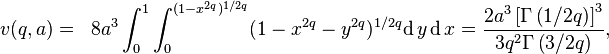\begin{align} v(q,a) & = & 8 a^3 \int_{0}^1 \int_{0}^{(1-x^{2q})^{1/2q}} (1-x^{2q}-y^{2q})^{1/2q} \mathrm{d}\, y \, \mathrm{d}\, x = \frac{2a^3\left[ \Gamma\left(1/2q\right) \right]^3}{3q^2\Gamma\left(3/2q\right)}, \end{align}

where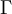$\Gamma$ is the Gamma function.

## Overlap algorithm

The most widely used overlap algorithm is on the basis of Perram and Wertheim method  .

## Phase diagram

The full phase diagram of hard superballs whose shape interpolates from cubes to octahedra was reported in Ref .